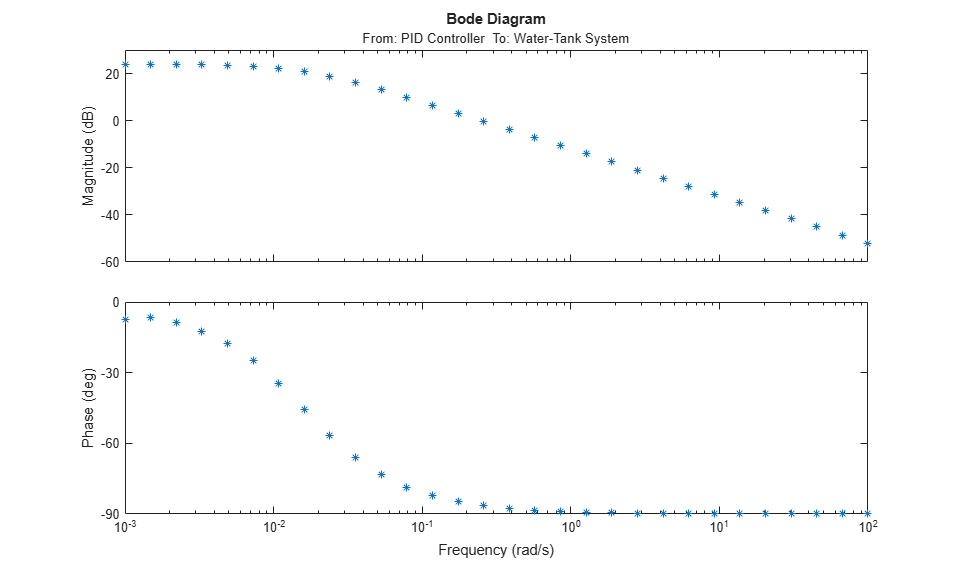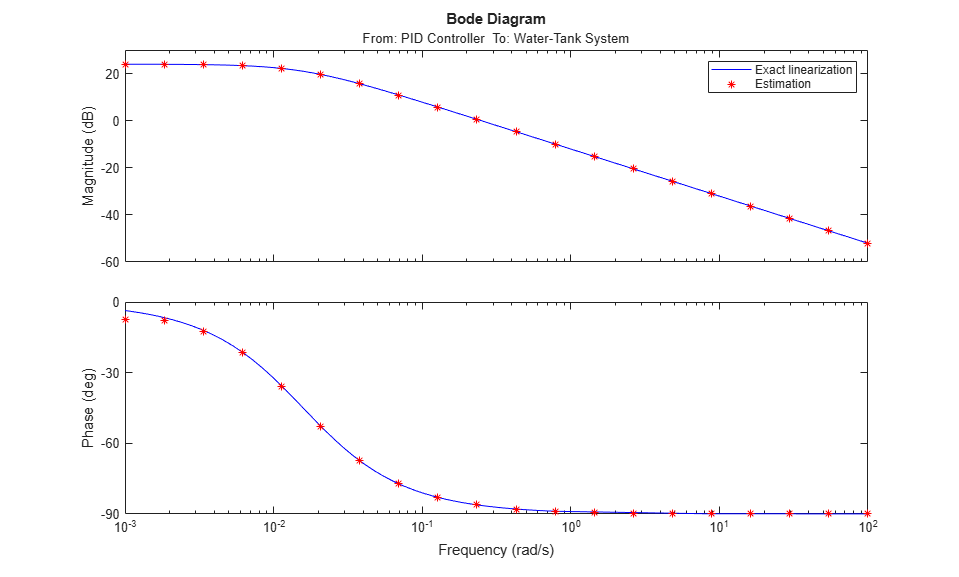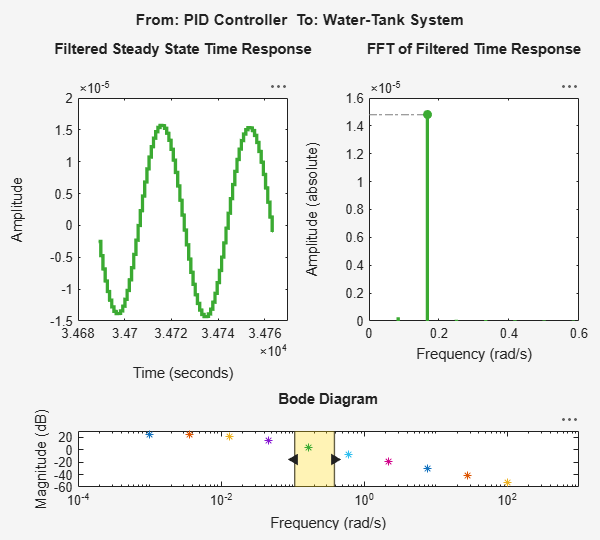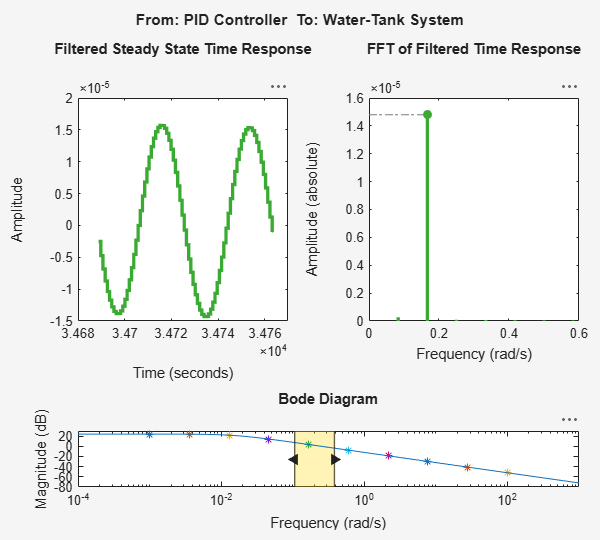# frestimate

Frequency response estimation of Simulink models

## Syntax

``sysest = frestimate(model,io,input)``
``sysest = frestimate(model,op,io,input)``
``[sysest,simout] = frestimate(model,op,io,input) ``
``[___] = frestimate(___,options)``
``sysest = frestimate(data,freqs,units)``

## Description

example

````sysest = frestimate(model,io,input)` estimates the frequency response of a Simulink® model using the specified input signal, the operating point defined by the model initial conditions, and the analysis points specified in `io`. ```
````sysest = frestimate(model,op,io,input)` initializes the model at the operating point `op` before estimating the frequency response. If the model initial conditions are not at steady state or not the operating point of interest, use this syntax to specify a different operating point.```

example

````[sysest,simout] = frestimate(model,op,io,input) `also returns the simulated model output. Use this syntax when you want to examine the estimation results using the Simulation Results Viewer (`frest.simView`).```
````[___] = frestimate(___,options)` computes the frequency response using additional options. You can use this syntax with any of the previous input and output argument combinations.```
````sysest = frestimate(data,freqs,units)` estimates the frequency response using simulation data obtained using the Frequency Response Estimator block in offline estimation mode. Use this syntax only with data logged using that block.```

## Examples

collapse all

Estimate the open-loop response of the plant in the `watertank` model. Open the model.

```model = 'watertank'; open_system(model) ```To estimate the open-loop response of the plant, define a linearization I/O set that specifies this portion of the model with analysis points. Define an input analysis point at the controller output, and define an open-loop output point at the plant output.

```io(1)=linio('watertank/PID Controller',1,'input'); io(2)=linio('watertank/Water-Tank System',1,'openoutput'); ```

Find a steady-state operating point for the estimation. For this example, use a steady-state operating point derived from the model initial conditions.

```watertank_spec = operspec(model); opOpts = findopOptions('DisplayReport','off'); op = findop(model,watertank_spec,opOpts); ```

Create an input signal for estimation. For this example, use a sinestream signal, which sends a series of separate sinusoidal perturbations at the frequencies you specify.

```input = frest.Sinestream('Frequency',logspace(-3,2,30)); ```

Estimate the frequency response of the specified portion of the model. The result is a frequency-response model containing responses at each of the frequencies specified in the sinestream signal.

```sysest = frestimate(model,op,io,input); size(sysest) ```
```FRD model with 1 outputs, 1 inputs, and 30 frequency points. ```

Examine the measured frequency response.

```bode(sysest,'*') ```Linearize a Simulink model and use frequency-response estimation to validate the exact linearization results.

Open the `watertank` model.

```model = 'watertank'; open_system(model); ```Obtain a linearization of the open-loop response of the plant. To do so, define the linearization I/O points, and find a steady-state operating point near the model initial conditions. Then, linearize the model.

```io(1)=linio('watertank/PID Controller',1,'input'); io(2)=linio('watertank/Water-Tank System',1,'openoutput'); watertank_spec = operspec(model); opOpts = findopOptions('DisplayReport','off'); op = findop(model,watertank_spec,opOpts); syslin = linearize(model,op,io); ```

To check the linearization, use the same analysis points and operating point to estimate the frequency response. For this example, use a sinestream input signal for the estimation.

```input = frest.Sinestream('Frequency',logspace(-3,2,20)); sysest = frestimate(model,op,io,input); ```

Compare the exact linearization and the estimated response in the frequency domain using a Bode plot.

```bode(syslin,'b-',sysest,'r*') legend('Exact linearization','Estimation') ```The Simulation Results Viewer lets you examine the results of frequency response estimation frequency by frequency. You open the viewer using the `frest.simView` command. To do so, store the simulation data using the `simout` output argument of `frestimate`.

Estimate the open-loop response of the plant in the `watertank` model. First, open the model.

```model = 'watertank'; open_system(model) ```Define a linearization I/O set that specifies the plant, and find a steady-state operating point for estimation.

```io(1)=linio('watertank/PID Controller',1,'input'); io(2)=linio('watertank/Water-Tank System',1,'openoutput'); watertank_spec = operspec(model); opOpts = findopOptions('DisplayReport','off'); op = findop(model,watertank_spec,opOpts); ```

Then, create an input signal for estimation, and estimate the frequency response of the specified portion of the model. Use the `simout` output argument to store the estimation data.

```input = frest.Sinestream('Frequency',logspace(-3,2,10)); [sysest,simout] = frestimate(model,op,io,input); ```

Open the Simulation Results Viewer.

```frest.simView(simout,input,sysest) ```The viewer shows you the steady-state time response and the FFT of that response for all frequencies within the range you select on the Bode Diagram section of the viewer. These plots can help you identify when the response deviates from the expected response. For more information about using the Simulation Results Viewer, see Analyze Estimated Frequency Response.

If you have a linear model of the system you are estimating, you can use the model as a baseline response for comparison in the viewer. For instance, you can compare a model obtained by exact linearization to the estimated frequency response. Use the linearization I/O set and the operating point to compute an exact linearization of the `watertank` plant.

```syslin = linearize(model,io,op); ```

Open the Simulation Results Viewer again, this time providing `syslin` as an input argument.

```frest.simView(simout,input,sysest,syslin) ```The Bode Diagram section of the viewer includes a line showing the exact response `syslin`. This view can be useful to identify particular frequencies where the estimated response deviates from the linearization.

## Input Arguments

collapse all

Simulink model, specified as a string or character vector. The model must be in the current working folder or on the MATLAB® path.

Analysis points set that contain inputs, outputs, and loop openings, specified as a linearization I/O object. The analysis point set defines the subset of the Simulink model whose frequency response you want to estimate. To create `io`:

• Define the inputs, outputs, and openings using `linio`.

• If the inputs, outputs, and openings are specified in the Simulink model, extract these points from the model using `getlinio`.

For frequency response estimation, I/O points cannot be on bus signals. `io` must correspond to the Simulink model `model` or a normal mode model reference in the model hierarchy. (If you use `frestimate` with an output analysis point in a model reference, the Total number of instances allowed per top model configuration parameter of the referenced model must be 1.)

Specifying I/O points for estimation is similar to specifying them for linearization. For more information on specifying linearization inputs, outputs, and openings, see Specify Portion of Model to Linearize.

Input signal for perturbing the model, specified as one of the following:

Operating point at which to initialize the model for estimation, specified as an `OperatingPoint` object created using one of the following functions.

Generally, you use a steady-state operating point for estimation. If you do not specify an operating point, the estimation process begins at the operating point specified by the model initial conditions. This operating point consists of the initial state and input signal values stored in the model.

Estimation options, specified as a `frestimateOptions` object. Available options include enabling parallel computing for estimation (requires Parallel Computing Toolbox™).

Response data logged for offline estimation using the Frequency Response Estimator block, specified as one of the following:

• A structure obtained by writing the data from the data output port of the block to the MATLAB workspace using a To Workspace block. The Save format parameter of the To Workspace block must be `Timeseries`.

• A `Simulink.SimulationData.Dataset` object obtained by using Simulink data logging to write the data at the data port to the MATLAB workspace.

For more information, see the data port description on the Frequency Response Estimator block reference page or Collect Frequency Response Experiment Data for Offline Estimation.

Frequencies for offline estimation, specified as a vector of positive values. When you collect response data using the Frequency Response Estimator block, you specify the frequencies for the estimation experiment using the Frequencies parameter of the block. Use the same vector of frequencies for `freqs` when you perform offline estimation with the logged data.

Units of frequencies for offline estimation, specified as one of the strings `"rad/s"` or `"Hz"` or one of the character vectors `'rad/s'` or `'Hz'`. When you collect response data using the Frequency Response Estimator block, you specify the units of the frequencies for the estimation experiment using the frequency units block parameter. Specify the same units when you perform offline estimation with the logged data.

## Output Arguments

collapse all

Estimated frequency response, returned as a frequency-response (`frd`) model object. The `frd` model has as many inputs and outputs as are specified in the linearization analysis points `io`.

The frequencies in `sysest` depend on what input signal you use for estimation, as follows:

• If you use a sinestream signal created with `frest.Sinestream`, the frequencies in `sysest` are the frequencies specified in the sinestream signal.

• If you use any other input signal, the frequencies are determined by the FFT computation that the function performs to extract the frequency response (see Algorithms).

If you use the `data` input argument to provide data collected using the Frequency Response Estimator block, then `sysest` is a SISO model. In this case, the frequencies in `sysest` are the frequencies you supply with the `freqs` input argument.

Simulation data collected during the estimation process, returned as a cell array of `Simulink.Timeseries` objects. The cell array has dimensions `m`-by-`n`, where `m` is the number of output points in the I/O set `io`, and `n` is the number of input points. This data can be useful for:

## Limitations

• If you use `frestimate` with an output analysis point in a model reference, the Total number of instances allowed per top model configuration parameter of the referenced model must be 1.

## Tips

• For multiple-input multiple-output (MIMO) systems, `frestimate` injects the signal at each input channel separately to simulate the corresponding output signals. The estimation algorithm uses the inputs and the simulated outputs to compute the MIMO frequency response. If you want to inject different input signals at the linearization input points of a multiple-input system, treat your system as separate single-input systems. Perform independent frequency response estimations for each linearization input point using `frestimate`, and concatenate your frequency response results.

## Algorithms

collapse all

`frestimate` injects the input signal you specify (uest(t)) at the input analysis points. It simulates the model and collects the response signal (yest(t)) at the output analysis points, as illustrated below for a sinestream input.In general, `frestimate` estimates the frequency response by computing the ratio of the fast Fourier transforms output signal and the input signal:

`$Resp=\frac{FFT\left({y}_{est}\left(t\right)\right)\text{\hspace{0.17em}}}{FFT\left({u}_{est}\left(t\right)\right)}.$`
• For sinestream input signals, the function discards the data collected during the specified settling periods of the signal at each frequency. (See Sinestream Input Signals.) If the filtering option of the sinestream signal is active, the function then applies a bandpass filter to the remaining signal at the corresponding frequency and discards one more period to remove any remaining transient signals. The function uses the FFT of the resulting signal to compute Resp. The resulting `frd` model contains all frequencies in the sinestream.

• For chirp input signals, the function discards any frequencies in the ratio Resp that fall outside the frequency range specified for the chirp. The resulting `frd` model contains all frequencies in the Fourier transform that fall within the chirp range.

• For other input signals, the resulting `frd` contains all the frequencies in the Fourier transform.

### Estimation Using Data from Frequency Response Estimator Block

You can use the `frestimate(data,freqs,units)` syntax to perform offline estimation with data from the Frequency Response Estimator block. In this case, `frestimate` uses the `Ready` field of the data structure to determine which data points to include the FFT computation of Resp.

• For sinestream mode, this signal indicates which periods to discard at each frequency, determined by the Number of settling periods block parameter.

• For superposition mode, this signal indicates which data falls within the data-collection window determined by the Number of periods of the lowest frequency used for estimation parameter.

`frestimate` interpolates Resp to generate the resulting `frd` model, which contains the frequencies you specified in the block experiment parameters. For more information, see the Frequency Response Estimator block reference page.

## Alternative Functionality

### App

Model Linearizer

Frequency Response Estimator

## Extended Capabilities

Introduced in R2009b